Page 3
Go to:  P1   P2     P4TERMINOLOGY: A coil can be used as a "pick-up" to detect magnetic or metal objects. It can be called a TRANSDUCER, Inductive transducer, magnetic transducer, coil, pick-up, inductor, magnetic coil, pickup coil, inductive loop, inductive sensor, magnetic sensor, metal detector, comparator, gold detector, crack detector - to detect cracks in welds etc, head - as in a tape recorder. It can have a core made of iron or ferrite and be called an INDUCTOR. It can have a moving iron core and be called a SOLENOID. An inductor can be used to smooth the ripple in a supply, or it can be used to generate a voltage higher than the supply and it can be wound on "poles" to create a motor. It can have an adjacent winding and become a transformer, isolator or step-up device. It can be a current transformer, or even a linear motor. And there are possibly dozens more  . . .

THE INDUCTOR AS A "PICK-UP."
Now we come to an important feature of a coil when it is used as a SENSOR.
When a magnet passes a coil (this includes the action of moving towards or away from a coil), a voltage is generated in the turns in the form of a sinewave.
We use the term "sinewave" to indicate the approximate shape of the waveform to distinguish it from other shapes such as "square wave," "exponential" or "glitch." A sinewave is a continuing waveshape with a gradual rise and fall as shown in the following example:The same type of waveform is produced if the magnet passes the end of the coil, into and out of the end of the coil or if the magnet passes through the centre.
If the magnet is moved rapidly, the amplitude of the waveform INCREASES (and there will be more "up's ad down's" in the same time-interval on the diagram above).
The amplitude is also determined by the strength of the magnet.There are three important points to note.
The first is the voltage produced by the coil as it passes the end of the coil.
When the magnet is directly opposite the end of the coil, the change in magnetic flux is zero and thus the voltage produced by the coil is zero.
The second point is the change in voltage produced by the coil. The output voltage changes from positive to negative during the very small portion of excursion when the magnet moves from a forward to reverse direction as seen by the end of the coil.
The third point is the coil produces zero voltage when the magnet is not moving.

If the magnet moves at a faster rate, the voltage produced by the coil will be greater as shown by the animation below: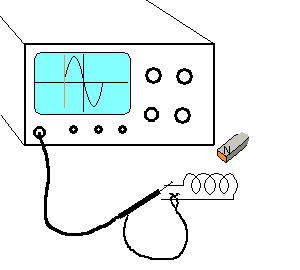If two or more coils are placed in close proximity with one or more coils providing a magnetic field, when a magnet or metal object is passed through the field, the  waveform produced by the sensing coil is altered. The size and shape may be very small but amplifying stages can produce amazing information.
This is the principle of a metal detector (gold detector) or a coin detector in a vending machine.
The resulting waveform can discriminate between a coin and a "slug," an aluminium can, a "pull ring" or a gold nugget. This type of detector is beyond this discussion. We will only be discussing how to detect the frequency of the pulses and the amplitude of the voltage from a single coil.

For an inductive pick-up to be successful, the magnet passing the end of the coil must be powerful and pass near to the end of the coil.
The output of a coil will generally be less than 700mV and must be AC coupled to a transistor so that the voltage produced by coil will modify the biasing of the transistor as shown in the diagram below: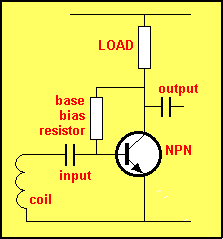In the diagram below, the magnet is passing the coil and when it is receding from the coil, the voltage produced by the coil turns the transistor OFF and the voltage on the collector rises.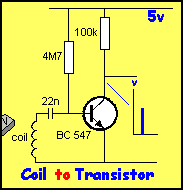The coil can be connected to a two-transistor arrangement to produce a square-wave output, suitable for circuits that require a noise-free signal: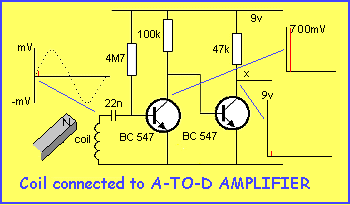A very simple circuit using a coil is shown in the diagram below. It is our METAL DETECTOR -1.
It uses a 16 turn coil (approx 6" dia) to detect metal objects such as coins etc. The circuit oscillates at approx 140kHz and the frequency produced is picked up by an AM radio to produce a quiet spot on the dial.  When a piece of metal is placed near the coil, the frequency of the circuit is altered and this is picked up by the radio as a low frequency tone. The circuit is extremely sensitive and a shift in frequency of as little as a few hertz will be clearly heard.ROTATION
A motor is an example of a rotating inductor. Most small motors are 3-pole (the minimum number of poles for self-starting) and if a motor is used as a generator, the output will correspond to the speed of rotation.
For a 3-pole motor, three waveforms are produced for each revolution and the following diagram shows the type of waveform produced: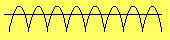If the motor increases in RPM, the waveform changes: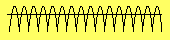A detecting circuit can be used to count the pulses and determine the RPM.
By putting fan blades on the shaft of a motor, you can measure wind velocity and the output of heating and cooling systems etc.
The output from the motor can be calibrated by placing the fan outside the window of a car traveling at a known velocity.

THE INDUCTOR AS A FILTER - also known as a CHOKE
When an inductor is placed in a circuit and reduces the ripple, as it does in the circuit below, it is also known as a CHOKE - it "chokes off" the ripple. This is old terminology and is called Jargon. (Jargon are words or sentences that are only understood by those who work in the particular field or "area" or job.)
We have already covered this feature at the beginning of this article but since it is so important, we will go over it again.
This time we will cover some of the features of a coil and one of its hidden "magical properties."  This magical property is the ability of a coil (inductor) to produce a BACK VOLTAGE or REVERSE VOLTAGE that opposes an increasing or decreasing voltage.
This is how the inductor smoothes (reduces) the ripple in the voltage emerging from the rectifier.
It all starts when a voltage increases (or decreases) in amplitude.
Any increasing voltage allows an increasing current to flow and this current increases the magnetic flux produced by the coil and this flux cuts all the other turns of the coil to produce a "back voltage."
This is the situation with the inductor "L" in the circuit below:When current flows, the voltage-waveform after the inductor, will be smaller than the waveform entering the inductor. The size of the waveform from the bridge will depend on the ripple from the transformer. The diagram above shows a waveform before and after the inductor but this is just a representation. It is not a true indication of the size of the waveform.
A power supply contains electrolytic called filter electrolytics and the diagram below shows one placed before the inductor and one after the inductor.This discussion is very complex because we are constantly discussing voltage waveforms then current waveforms. But this is the way it has to be.
The other thing that is hard to understand is the term "DC." When we say "DC" we mean a steady or unchanging voltage or current. Even though the real meaning of DC is "Direct Current" we still refer to an unchanging voltage as "DC." Again this is jargon and that's why you need to understand the meaning of the words.

The voltage waveform entering the inductor will actually be something like 10v DC with a ripple of say 300mV and 9.5v with a ripple of 50mV leaving the inductor.
This is shown on the diagram below: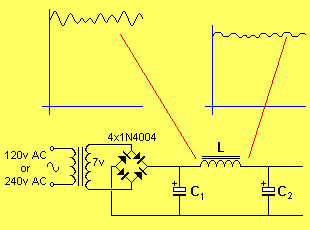The size of the "output" waveform (from the inductor) will depend on the inductance of L and the value of the load. If the load is an amplifier, the current will be changing all the time according to the level of music or speech. If the load is a globe, we call the load a "steady load." By this we mean the current is steady.
Even if you are supplying a steady load such as a globe, the waveform (ripple) entering the inductor will create a "back-voltage" in the inductor that will reduce the peaks and prevent the lows getting any lower. This is due to the input waveform having a "ripple component." If the input voltage was "pure DC," the inductor would not be needed. This is just an example of the operation of the inductor. There will be a voltage drop across the indicator due to the resistance of the winding. This can be proven by connecting the inductor to a battery (such as a car battery) and measuring the voltage drop across it for any given load.
Now we go back to our example: The output ripple is not constant. As the current is increased, the output ripple will increase. This is due to a number of factors.
The transformer will not be able to supply the higher current and its output voltage will drop. The electrolytics will provide less filtering at the higher current (see circuit below) and the inductor will become "magnetically saturated" and not produce the same filtering. All these characteristics will combine to produce a varying ripple voltage on the output.
There is another factor to consider.
You simply cannot select an inductor by inductance alone. For instance, you cannot simply say "use a 10,000uH choke." Not all 10,000uH chokes are the same. Here is an example of 3 different 10,000uH chokes.
But before we do, remember this:

Ten thousand microhenries is the same as 10 millihenries.

1,000nH = 1
µH
1,000
µH = 1mH
1,000mH = 1Henry

The following photos show different types of chokes. All have the same inductance (10,000uH) but they will all produce a different output because they have different coil-resistances. The second inductor seems to be up-side-down, but the two ends are soldered to the PC board. It is a surface-mount item.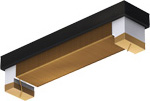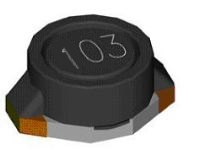10milliHenry  2R7 Max current 3A 10milliHenry 15R Max current 2mA 10milliHenry  33R Max current 20mA

The photos above do not show the real size of the components but you can see each has a maximum current rating.
The current rating is the dominant factor.
That's why a 10mH choke can have so many different sizes.

THE INDUCTOR IN A POWER SUPPLY
An inductor can be used in a power supply to reduce the ripple.
In most power supplies this is done with electrolytics, however we will explain how the inductor operates.
The inductor only operates on the fluctuating part of the voltage.
If the voltage rises, it increases the magnetic flux and this is called expanding flux. This flux cuts all the turns of the coil and produces a voltage in the turns that is opposite to the incoming voltage.
Thus the increasing voltage does not enter the inductor.
This is how the inductor reduces the ripple on the output.
In the following circuit, the output has a reduced ripple due to the inductor:The following diagram shows an inductor with a closed magnetic circuit. The "magnetic circuit" is shown via the arrows. And an inductor with an AIR GAP.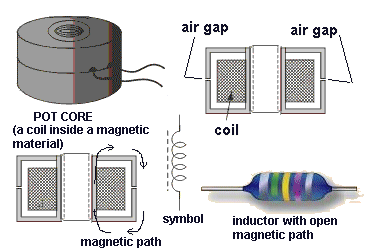An "open" magnetic circuit is shown above via the colour-coded inductor. The "magnetic circuit" is the core but the flux does not form a continuous loop. This type of magnetic circuit is very inefficient and has high losses. But it is quite acceptable if the magnetic flux is kept to a low level where the core is not saturated.

DESIGNING AN  INDUCTOR AS A FILTER (choke)
Designing an inductor for the above application is a very complex mathematical problem. We do not know the ripple on the input to the inductor or any of the voltage values as a small transformer as show in the diagram has a very poor regulation factor and the output can be up to 60% higher than the stated output so that the voltage drops to the required level on full output current.
The best thing is to have a range of inductors and try the
m.
If you are winding your own inductor it is best to add too many turns to a core and gradually remove them as needed.
Nothing beats "actual applications" as there are too many variables to be able to design something from a chart.
If you don't know where to start, look at a power supply. Remember the frequency of this power supply is either 50Hz or 60Hz. It is not a 40kHz design. The inductance will be much higher than for a 40kHz circuit.
The same approach is recommended for any type of inductor.
An inductor is very difficult to design.
Projects do not always consume a constant current and the ripple present on the unfiltered side of a power supply needs to be viewed on a CRO.
Rather than using complex mathematical formulae to work out the value of an inductor, the easiest way is to try different values.

A choke in a DC circuit has to have an AIR GAP. That's why it is called a CHOKE. This is to differentiate it from an INDUCTOR.
In a DC circuit  (one that has ripple) voltage will be present all the time and current will be flowing all the time. This means the choke will be producing magnetic flux all the time and this flux will SATURATE the magnetic core. This means an ordinary inductor will be worthless. But if a sheet or cardboard is fitted between the top and bottom sections of the magnetic core before it is dipped into a sealing compound to prevent the wire and other parts vibrating when using the component, the cardboard will produce an AIR GAP.
When you fit the component to a circuit it will produce magnetic flux and all this flux will be lost in the air gap. The air gap actually has to be the right width but 0.1mm will be a good start.
This means the current flowing through the device will produce magnetic flux and it will all be lost in the air gap and when additional current flows (due to the input voltage rising) the extra flux will be delivered to the magnetic circuit.
When the input voltage reduces, the magnetic flux will collapse and pass into all the turns of the winding and produce a voltage that will add to the lower voltage and the output voltage will be slightly increased.
The choke prevents the voltage rising and prevents the voltage dropping. There are limits to this and if you want something to reduce a 1v ripple at 1 amp, the choke will weight say 100gm and be quite expensive.
This can be done with electrolytics that are much smaller and much cheaper.
Chokes were originally used because they came as part of a speaker field coil and size and weight did not matter.
But today's technology with switch-mode power supplies and miniaturisation means high-frequency operation and size and weight and cost all point towards using electrolytics.

THE WIRE
The size of the wire (the thickness) is an important factor when designing an inductor.
It is always best to use the thickest wire for the application.

WHY?
The thickest wire produces the best result.
But finding the best thickness (gauge) is a complex problem.
Let's explain:
In some cases the wire-size is easy to work out. For a transformer, the size of the former (the plastic bobbin on which the wire is wound) and the area (space for the winding) is already known. You will also know the number of turns required for each volt and thus the total number of turns will be known. The size (gauge) of wire can be found from a table.

But an electromagnet (or solenoid) is different. This is basically a DC component, although it will work on AC (say 50Hz or 60Hz).
These devices are designed to produce magnetic flux (to pull in an armature or core or collect scrap metal - such as in a metal-yard) and the flux is produced by multiplying the number of turns by the current flowing though the coil. This is called AMP-TURNS.
Theoretically, the same flux can be produced by one turn and 100amps or 100 turns and one amp or 500 turns and 200mA.
But the current will depend on the gauge of the wire (as thin wire has a higher resistance - and this will limit the amount of current that will flow).
So, the maximum flux will depend on selecting a wire-size. Working out how many turns can be fitted onto the former (wound on the bobbin) and thus the total length of wire can be determined. This will give you the resistance of the coil and thus the current (use a table to find the resistance and Ohm's Law to find the current).  Multiply the number of turns by the current. Select another gauge of wire and eventually you will get a table of values. From this table you will see a maximum value.

If you are designing an inductor for an AC voltage or any fluctuating or changing voltage, you will utilizing the coils: "magic property." This is the fact that the expanding or collapsing flux from each turn cuts all the other turns of the coil to produce a voltage in the opposite direction. This is called the INDUCTANCE of the coil and it means the coil does not only produce magnetic flux as described in the electromagnet or solenoid above, but it also has an effect of limiting or reducing the current you can deliver to the coil. This is because a coil, solenoid or inductor always limits or reduces the current at the instant you apply the voltage.
If you are constantly utilizing this feature by supplying a rising and falling voltage, the coil will behave completely differently to a solenoid (that has a DC supply connected to it for a long period of time).
This means you must treat the coil completely differently to a solenoid.
It will produce completely different results, however it is best to use the thickest gauge available.

WHY?
The thick wire allows more of the flux to pass through the adjacent turns of the winding and thus produce a higher reverse voltage.
In addition, the winding will have a lower resistance and this will allow a higher current to flow and thus create more flux.

THE INDUCTOR IN "FLYBACK"
We mentioned above, one of the "magical properties" of an inductor is its ability to produce a "back-voltage" or "reverse voltage."
There is an even-more-magical extension to this.
If a voltage is applied to an inductor and then removed, the back-voltage will be VERY HIGH. It can be 100 times (or more) higher than the applied voltage.
This is the principle of the ignition system in a car. The distributor connects the ignition-coil to the battery of the car via the points and then the points open.
The collapsing magnetic filed in the ignition-coil is passed from the end of the coil to the distributor rotor-cap, and this is the rotating part of the distributor. It sends the 20,000v to the appropriate spark-plug.
So, the collapsing property of an inductor has been known for a long time and it has also been used in electronics for many applications.
Uses such as electric fences, high voltage generators, EHT circuits in TV's, switch-mode power supplies.
This back-voltage is also produced by relays, motors, door-latches and where-ever a coil is energised.
In most cases this voltage is higher than the operating voltage of the components in the circuit and it must be prevented from damaging them.
In the case of relay, the back-emf, (this is what the back-voltage is called) is snubbed (reduced) by the diode. The back-voltage has the opposite polarity to the supply and this voltage is placed directly across the diode and it forms a very low resistance path to absorb the energy.Another way to prevent the high voltage damaging the circuit is to provide high-voltage insulation and shielding. Apart from spike-suppression capacitors across the chips, there is no other way to prevent high voltages damaging a circuit.

THE INDUCTOR and the word "IMPEDANCE"
When we talk about the value of an INDUCTOR we say: What is the IMPEDANCE of the inductor?  In other words we are asking: What is the effect of the inductor having on the circuit?
In other words, if we replace the inductor with a resistor, what is the value of the resistor?
The answer is its INDUCTIVE REACTANCE and is symbolized by the letter "X" and is measured in ohms, just like a resistance.
When an inductor is placed in a circuit that contains a waveform, the inductor produces a back voltage and this voltage increases as the frequency increases. This means the inductor presents a changing effect, according to the frequency and its INDUCTIVE REACTANCE changes.
You cannot measure the value with a multimeter.  You have to work it out by taking other values, such as measuring the frequency and the impedance of the coil.
Using the formula:

XL = 2πfL

we can find the reactance at any particular frequency.

THE INDUCTOR in SWITCH-MODE POWER SUPPLIES
Switch-Mode power supplies are everywhere in electronics.
Since the introduction of high-voltage transistors, these type of supplies are now found in "plug-packs," (wall-worts) and all types of phone chargers.
But the question is: How do you work out the value of the inductor. Firstly you want the inductor to be as small as possible and as cheap as possible.
Firstly you have to know the current that will be passing in the circuit. This will give the gauge of the wire.
Next you have to know the pulses into the inductor will be 2 or more times the average current that will be passing through the circuit.  This is because the inductor will be passing twice the current for half the time as the remaining 50% of the time it will be delivering current to the output part of the circuit.
Now, the next part is technical. It takes a very short period of time to pulse the inductor and generate the maximum magnetic field. If you pulse it for longer than this time, the energy is wasted in excess magnetic flux that will not be converted to energy when you cease the pulse.
We have chosen an inductance with a very low inductance to keep the size very small and it will only take a few microseconds to fully energise it.  This means the circuit must be operating at a very high frequency to keep the "charge-time" below the time needed to fully energise the inductor.
If you supply energy to an inductor during the time when it is producing magnetic flux, it appears as a very high resistance - may be 10 or 20 times the actual resistance of the coil. The "winding" may have a resistance as low as 0.1 ohms or maybe 1 ohm but it will appear as a resistance of maybe 10 ohms. But when the inductor eventually produces the maximum flux (this is when the magnetic circuit is saturated) the circuit sees the inductor as 0.1 ohm and it can easily blow-up the driving transistor.  That's why you have to get the timing correct.
[When you are "charging" an inductor  - in other words, when you are supplying energy to an inductor and increasing the magnetic field, the current through each turn is called  EXPANDING FLUX and it is cutting all the other turns and producing a microscopic voltage in each turn that is opposite to the voltage you are supplying and this means you maybe supplying 10v and the back voltage is 9v. This effectively means you are supplying only 1v and thus only 1/10th the current will be allowed to pass through the inductor. That's how  the inductor appears to have ten times the resistance.]
Now back to the topic:
That's how we get operating frequencies of 40kHz or 400kHz and even 3MHz. These are all of a duration that prevents saturation. The "ON-TIME" is slightly less than the time required to saturate the inductor.
The inductor is actually used in FLY-BACK mode in many circuits where the circuit is turned off very quickly and the magnetic flux produced by the inductor collapses very quickly and is passed to the receiving circuit.
The inductor actually produces a very very high voltage at a low current but the magic of magnetism will convert this very very high voltage into a voltage determined by the receiving circuit (the receiving circuit limits the voltage to a pre-determined value) and the current will rise to a value so that the input energy will be delivered to the output (less a few percent losses). You simply multiply the volts and current and time to get the input energy and this will be the output energy. It is in the form of about 50% ON and 50% OFF.
This FLY-BACK phenomenon is only produced if the supply to the inductor is turned off very quickly (instantaneously). If you turn off the supply slowly, the inductor will output a reverse voltage but its value will be governed by the device that is lowering the voltage (such as the driver transistor and the high-voltage phenomenon of flyback will not be produced. The magnetic field will just "fall-back" into the turns and be determined by the driver transistor as the transistor will still have an effect on the current flowing in the circuit.
So, how do you select an inductor.
Look at other circuits and see what value they have used.
Alternatively buy a set of inductors and try each one.  Use a weak battery to drive the circuit and test the output. You will see a gradual rise in performance and then a lowering in performance.
Choose the peak performance and drive the circuit with a better power-supply.
You don't need any mathematics, calculations or skill.  Just experimentation. That's how these circuits were developed in the first place. EXPERIMENTATION.

THE SECRET
The following circuit uses an inductor to separate the 3.6v across a 1 watt LED from a 15v power supply.
This circuit explains the secret: "HOW AN INDUCTOR WORKS."
The resistance of the inductor is less than one ohm and it sees about 14v on the left lead and 3.6v on the right lead.
So . . . how does it work?
How does it allow 14v to be present on one side and 3.6v on the other side. The 1R resistor between the inductor and LED only has about 0.3v across it.
Obviously the resistance of the inductor is not creating the 14 - 3.6 = 10.4volts across the leads.
It must be something to do with the turns of wire on the ferrite core.
On the right lead of the inductor is a 100u electrolytic. This capacitor has the effect of preventing the voltage rising or falling. It keeps the voltage fixed and stable.
When the BC327 transistor turns ON, it sends a pulse of current to the left lead of the inductor. This current enters the inductor and produces magnetic flux that cuts all the turns of wire and produces a voltage in the opposite direction.
This voltage is nearly the same value as the voltage entering the coil and the result is very little current will flow though the inductor.
This current is exactly the correct amount to fully illuminate the LED.
The inductor can only prevent too much current flowing FOR A VERY SHORT PERIOD OF TIME.
That's why the oscillator is a very HIGH FREQUENCY.
That's the secret behind the operation of the inductor.
It creates a voltage across it that limits the current flowing through the turns.
Of course the inductor must be selected to have the correct number of turns and the correct wire-size and correct ferrite-size.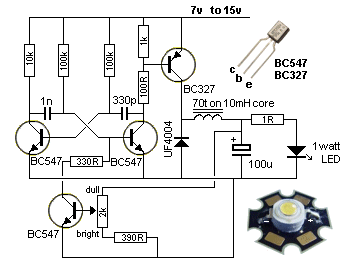CONCLUSION
This discussion has covered only a fraction of the mysteries of the inductor. There are special ways to wind an inductor for high voltage circuits. There are special ways to reduce the inductance and there are special cores to increase the inductance.
There are ways to tap an inductor to get all sorts of results such as high current, high impedance, low impedance and impedance-matching. You can add a second, third and fourth winding to get isolation, impedance-matching, high voltage or high current. Inductors can be made long, short, fat or any shape to fit onto a circuit.
That's why there are so many shapes, sizes and pin-outs.

To understand "the Inductor" you need to make a simple circuit that you can adjust and change the physical properties of the inductor.
The following circuit is a perfect example for experimenting. It uses a single 1.5v cell to illuminate a white LED. We know a white LED requires at least 3.2v for it to illuminate, so the circuit is "magically" increasing the 1.5v to a higher voltage.
The coil (the two coils) is actually a transformer and it is acting in FLYBACK MODE.
It has no core (just air) and you can separate the turns and see what effect it has on the operation.

BIKE FLASHER - Amazing!
This bike flasher uses a single transistor to flash two white LEDs from a single cell. And it has no core for the transformer - just AIR!
All Joule Thief circuits you have seen, use a ferrite rod or toroid (doughnut) core and the turns are wound on the ferrite material. But this circuit proves the collapsing magnetic flux produces an increased voltage, even when the core is AIR. The fact is this: When a magnetic filed collapses quickly, it produces a higher voltage in the opposite direction and in this case the magnetic field surrounding the coil is sufficient to produce the energy we need.
Wind 30 turns on 10mm (1/2" dia) pen or screwdriver and then another 30 turns on top. Build the first circuit and connect the wires. You can use 1 or two LEDs. If the circuit does not work, swap the wires going to the base.
Now add the 10u electrolytic and 100k resistor (remove the 1k5). The circuit will now flash. You must use 2 LEDs for the flashing circuit.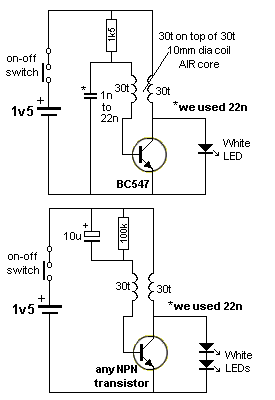THE IMPROVED BIKE FLASHER CIRCUIT

The original 30 turns + 30 turns coil is shown on the right. The circuit took 20mA to illuminate two LEDs.
The secret to getting the maximum energy from the coil (to flash the LEDs) is the maximum amount of air in the centre of the coil. Air cannot transfer a high magnetic flux so we provide a large area (volume) of low flux to provide the energy. The larger (20mm) coil reduced the current from 20mA to 11mA for the same brightness. This could be improved further but the coil gets too big. The two 30-turn windings must be kept together because the flux from the main winding must cut the feedback winding to turn ON the transistor HARD.
When the transistor starts to turn on via the 100k, it creates magnetic flux in the main winding that cuts the feedback winding and a positive voltage comes out the end connected to the base and a negative voltage comes out the end connected to the 100k and 10u. This turns the transistor ON more and it continues to turn ON until fully turned ON. At this point the magnetic flux is not expanding and the voltage does not appear in the feedback winding.
During this time the 10u has charged and the voltage on the negative lead has dropped to a lower voltage than before. This effectively turns off the transistor and the current in the main winding ceases abruptly. The magnetic flux collapses and produces a voltage in the opposite direction that is higher than the supply and this is why the two LEDs illuminate. This also puts a voltage through the feedback winding that keeps the transistor OFF. When the magnetic flux has collapsed, the voltage on the negative lead of the 10u is so low that the transistor does not turn on. The 100k discharges the 10u and the voltage on the base rises to start the next cycle.
You can see the 100k and 1k5 resistors and all the other parts in a "birds nest" to allow easy experimenting.
Note: Changing the turns to 40t for the main winding and 30t for the feedback (keeping the turns tightly wound together by winding wire around them) reduced the current to 8-9mA.

Go to:  P4

2/07/22